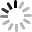# 提升網站品質，擁抱 Javascript ES6## 有用到 ES6 的地方

### 1. 改用 fetch

``````fetch('XXXX.json')
.then(response => {
return response.json();
}).then(result => {
return result;
});
``````

### 2. 使用 async、 await

async 和 await 是 promise 的語法糖，在主流瀏覽器已經全部支援的狀況下，沒道理不採用這麼好用的新語法，由於上述 fetch 是 promise 物件，所以透過 async 和 await，就能再確認資料載入後，再進行後續的事情 ( 在畫面上產生 DOM、隨機取出文章...等 )。

``````!async function(){
const list = () => {
return fetch('XXXX.json')
.then(response => {
return response.json();
}).then(result => {
return result;
});
};
const data = await list();
// do something...
}();
``````

### 3. 使用 const、let 和箭頭函數

``````// 原本寫法
var a =  function(){ ... }

// 改成箭頭函式
const a = () => { ... }
``````

### 4. 使用 every 處理陣列

every 是 ES6 語法裡處理陣列的好方法，有別於 forEach 無法停止 ( 迴圈一定得跑完才會停 )，every 可以撰寫邏輯判斷，只要 return false 就會停止迴圈，加速，加快陣列的操作和運算。

``````const arr = [...];
arr.every(e => {
if(...){
return false;
}else{
return true;
}
});
``````

### 5. 使用模板字符串 Template Literal

``````// 原本寫法
var a = 'oxxo';
var b = 'xoox';
var c = a + '你是不是' + b + '呀？';

// 改成模板字符串
var a = 'oxxo';
var b = 'xoox';
var c = `\${a}你是不是\${b}呀？`;
``````